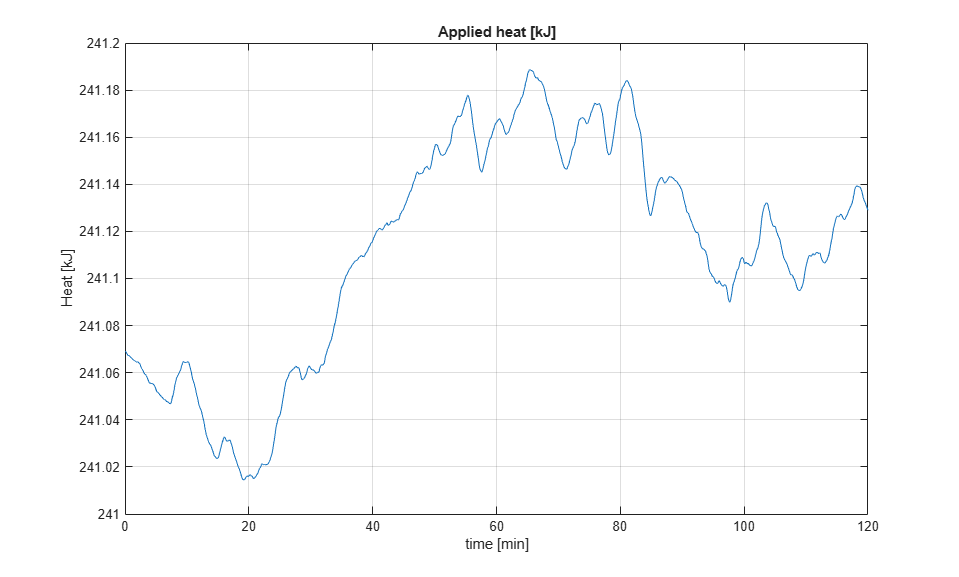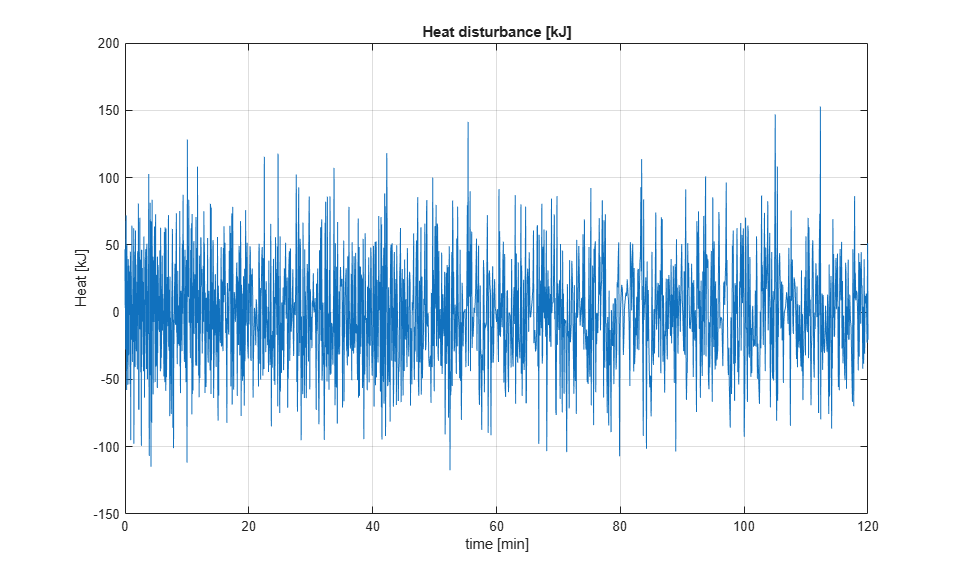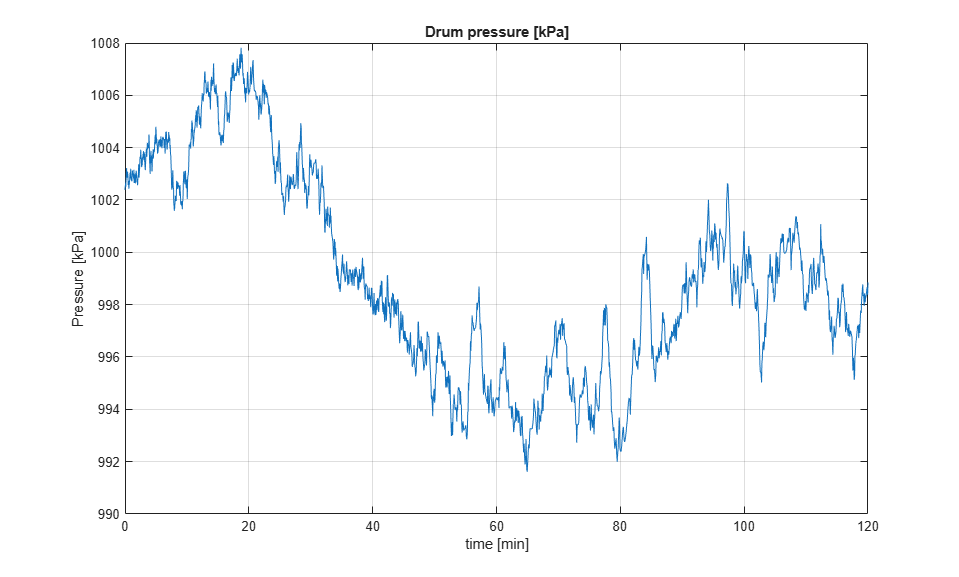# Regulate Pressure in Drum Boiler

This example shows how to use Simulink® Control Design™ software, using a drum boiler as an example application. Using the operating point search function, the example illustrates model linearization as well as subsequent state observer and LQR design. In this drum-boiler model, the control problem is to regulate boiler pressure in the face of random heat fluctuations from the furnace by adjusting the feed water flow rate and the nominal heat applied. For this example, 95% of the random heat fluctuations are less than 50% of the nominal heating value, which is not unusual for a furnace-fired boiler.

### Open the Model

```mdl = 'Boiler_Demo'; open_system(mdl) ```When you open boiler control model the software initializes the controller sizes. `u0` and `y0` are set after the operating point computation and are therefore initially set to zero. The observer and regulator are computed during the controller design step and are also initially set to zero.

### Find Nominal Operating Point and Linearize Model

The model initial state values are defined in the Simulink model. Using these state values, find the steady-state operating point using the `findop` function.

Create an operating point specification where the state values are known.

```opspec = operspec(mdl); opspec.States(1).Known = 1; opspec.States(2).Known = 1; opspec.States(3).Known = [1;1]; ```

Adjust the operating point specification to indicate that the inputs must be computed and that they are lower-bounded.

```opspec.Inputs(1).Known = [0;0]; % Inputs unknown opspec.Inputs(1).Min = [0;0]; % Input minimum value ```

Add an output specification to the operating point specification; this is necessary to ensure that the output operating point is computed during the solution process.

```opspec = addoutputspec(opspec,[mdl '/Boiler'],1); opspec.Outputs(1).Known = 0; % Outputs unknown opspec.Outputs(1).Min = 0; % Output minimum value ```

Compute the operating point, and generate an operating point search report.

```[opSS,opReport] = findop(mdl,opspec); ```
``` Operating point search report: --------------------------------- opreport = Operating point search report for the Model Boiler_Demo. (Time-Varying Components Evaluated at time t=0) Operating point specifications were successfully met. States: ---------- <strong>Min</strong> <strong>x</strong> <strong>Max</strong> <strong>dxMin</strong> <strong>dx</strong> <strong>dxMax</strong> <strong>___</strong> <strong>___</strong> <strong>___</strong> <strong>_____</strong> <strong>___________</strong> <strong>_____</strong> (1.) Boiler_Demo/Boiler/Steam volume 5.6 5.6 5.6 0 7.8501e-13 0 (2.) Boiler_Demo/Boiler/Temperature 180 180 180 0 -5.9262e-14 0 (3.) Boiler_Demo/Observer/Internal 0 0 0 0 0 0 0 0 0 0 0 0 Inputs: ---------- <strong>Min</strong> <strong>u</strong> <strong>Max</strong> <strong>___</strong> <strong>__________</strong> <strong>___</strong> (1.) Boiler_Demo/Input 0 2.4107e+05 Inf 0 100.13 Inf Outputs: ---------- <strong>Min</strong> <strong>y</strong> <strong>Max</strong> <strong>___</strong> <strong>______</strong> <strong>___</strong> (1.) Boiler_Demo/Boiler 0 1002.4 Inf ```

Before linearizing the model around this point, specify the input and output signals for the linear model. First, specify the input points for linearization.

```Boiler_io(1) = linio([mdl '/Sum'],1,'input'); Boiler_io(2) = linio([mdl '/Demux'],2,'input'); ```

Next, specify the open-loop output points for linearization.

```Boiler_io(3) = linio([mdl '/Boiler'],1,'openoutput'); setlinio(mdl,Boiler_io); ```

Find a linear model around the chosen operating point.

```Lin_Boiler = linearize(mdl,opSS,Boiler_io); ```

Finally, using the `minreal` function, make sure that the model is a minimum realization.

```Lin_Boiler = minreal(Lin_Boiler); ```
```1 state removed. ```

### Design Regulator and State Observer

Using this linear model, design an LQR regulator and Kalman filter state observer. First, find the controller offsets to make sure that the controller is operating around the chosen linearization point by retrieving the computed operating point.

```u0 = opReport.Inputs.u; y0 = opReport.Outputs.y; ```

Now, design the regulator using the `lqry` function. Tight regulation of the output is required while input variation should be limited.

```Q = diag(1e8); % Output regulation R = diag([1e2,1e6]); % Input limitation [K,S,E] = lqry(Lin_Boiler,Q,R); ```

Design the Kalman state observer using the `kalman` function. For this example, the main noise source is process noise. The noise enters the system only through one input, hence the form of `G` and `H`.

```[A,B,C,D] = ssdata(Lin_Boiler); G = B(:,1); H = 0; QN = 1e4; RN = 1e-1; NN = 0; [Kobsv,L,P] = kalman(ss(A,[B G],C,[D H]),QN,RN); ```

### Simulate Model

Simulate the model for the designed controller.

```sim(mdl) ```

Plot the process input and output signals. The following figure shows the feed water actuation signal in kg/s.

```plot(FeedWater.time/60,FeedWater.signals.values) title('Feedwater flow rate [kg/s]'); ylabel('Flow [kg/s]') xlabel('time [min]') grid on ```The following plot shows the heat actuation signal in kJ.

```plot(Heat.time/60,Heat.signals.values/1000) title('Applied heat [kJ]'); ylabel('Heat [kJ]') xlabel('time [min]') grid on ```The next figure shows the heat disturbance in kJ. The disturbance varies by as much as 50% of the nominal heat value.

```plot(HeatDist.time/60,HeatDist.signals.values/1000) title('Heat disturbance [kJ]'); ylabel('Heat [kJ]') xlabel('time [min]') grid on ```The figure below shows the corresponding drum pressure in kPa. The pressure varies by about 1% of the nominal value even though the disturbance is relatively large.

```plot(DrumPressure.time/60,DrumPressure.signals.values) title('Drum pressure [kPa]'); ylabel('Pressure [kPa]') xlabel('time [min]') grid on bdclose(mdl) ```